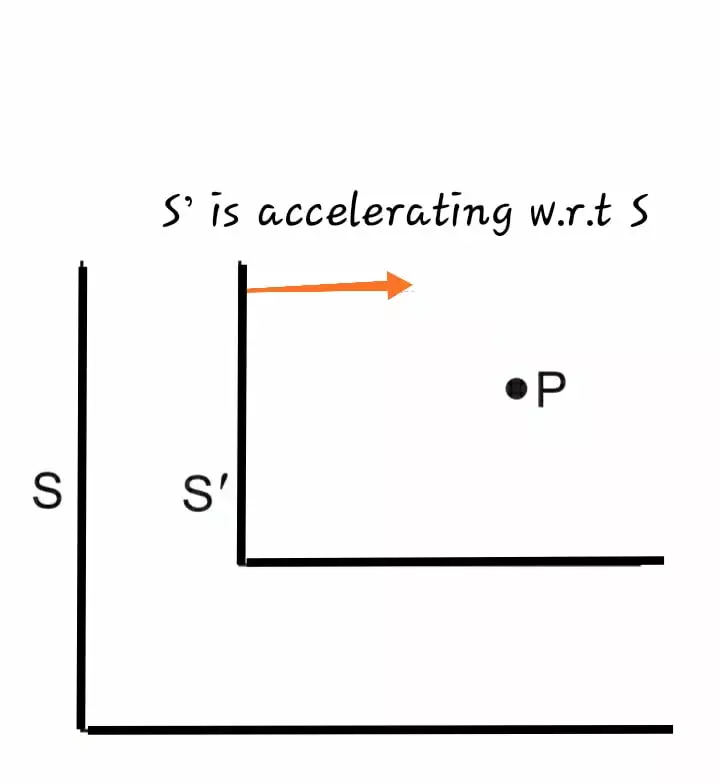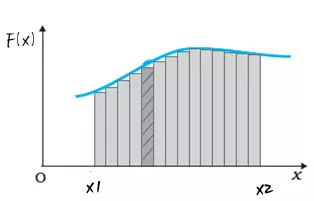# Category Physics Class – 11

## Derivation of work energy theorem class 11 | 2 cases rotational and translationalWork-Energy Theorem Today, we are going to derive the work-energy theorem and its derivation is universally applicable for all constant and variable forces and for translation and rotational work. Work-Energy Theorem For translation work Work Energy theorem in some books…

## Frame of reference and pseudo force | definition, meaning, concepts, formula.The frame of reference and pseudo force In Physics what we do is observe Nature, different Phenomenon and describe our observations, i.e where the event happened when the event happened and this all depends on different observers. For example: suppose…

## Conservative and non conservative forces and Potential energy Class 11Conservative and non conservative forces In this article, we will discuss conservative and non conservative forces and think about potential energy and its definition,  and we will learn about What are Conservative and Non conservative forces conservative field theory. so…

## Work done : basic meaning, Class 11 core concepts, work done by force, unit, factors affecting work doneIntroduction In our daily life we use the word “work done” when we do something like we push a car for some distance , we pull our bag or luggage  ,we lift something from ground floor to upper floors and…